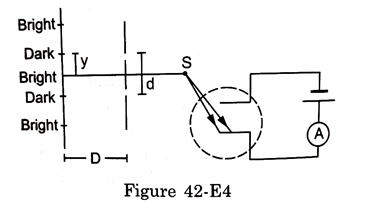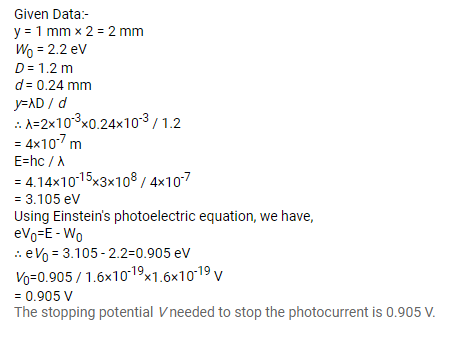# In the arrangement shown in the figure,

Question:

In the arrangement shown in the figure, $y=1.0 \mathrm{~mm}, d=0.24 \mathrm{~mm}$ and $D=1.2 \mathrm{~m}$. The work function of the material of the emitter is $2.2 \mathrm{eV}$. Find the stopping potential $V$ needed to stop the photocurrent.

Figure 42-E4Solution: